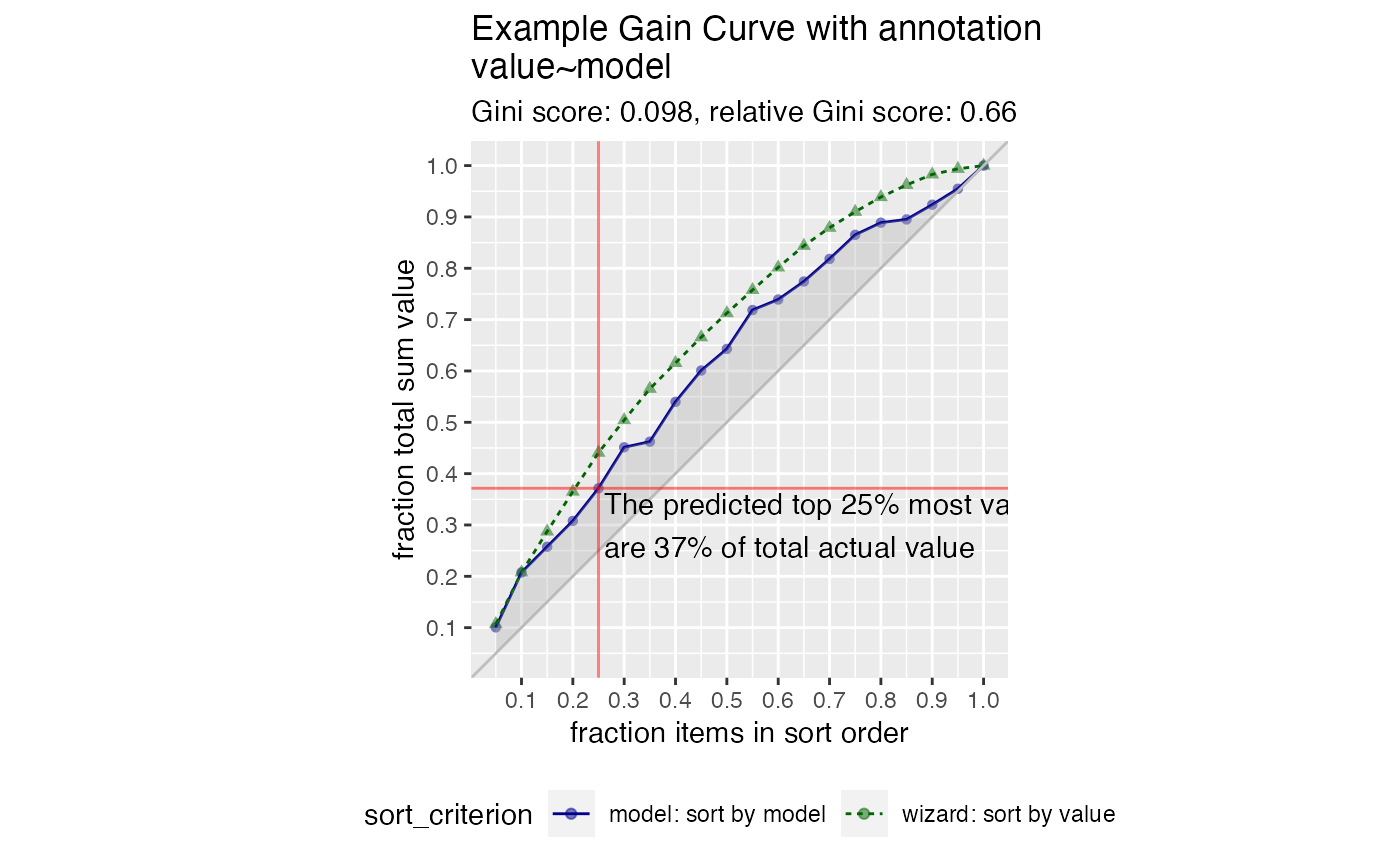Plot the cumulative gain curve of a sort-order with extra notation.

GainCurvePlotWithNotation(
frame,
xvar,
truthVar,
title,
gainx,
labelfun,
...,
estimate_sig = FALSE,
large_count = 1000,
model_color = "darkblue",
wizard_color = "darkgreen",
crosshair_color = "red",
text_color = "black"
)

## Arguments

frame data frame to get values from name of the independent (input or model score) column in frame name of the dependent (output or result to be modeled) column in frame title to place on plot the point on the x axis corresponding to the desired label a function to return a label for the marked point no unnamed argument, added to force named binding of later arguments. logical, if TRUE compute significance numeric, upper bound target for number of plotting points color for the model curve color for the "wizard" (best possible) curve color for the shaded area under the curve color for the annotation location lines color for the annotation text

## Details

This is the standard gain curve plot (see GainCurvePlot) with a label attached to a particular value of x. The label is created by a function labelfun, which takes as inputs the x and y coordinates of a label and returns a string (the label).

GainCurvePlot

## Examples


set.seed(34903490)
y = abs(rnorm(20)) + 0.1
x = abs(y + 0.5*rnorm(20))
frm = data.frame(model=x, value=y)
gainx = 0.10  # get the top 10% most valuable points as sorted by the model
# make a function to calculate the label for the annotated point
labelfun = function(gx, gy) {
pctx = gx*100
pcty = gy*100

paste("The top ", pctx, "% most valuable points by the model\n",
"are ", pcty, "% of total actual value", sep='')
}
WVPlots::GainCurvePlotWithNotation(frm, "model", "value",
title="Example Gain Curve with annotation",
gainx=gainx,labelfun=labelfun)